# Data analysis using Pandas

Pandas are the most popular python library that is used for data analysis. It provides highly optimized performance with back-end source code purely written in C or Python

We can analyze data in Pandas with:

## Pandas Series

Series in Pandas is one dimensional(1-D) array defined in pandas that can be used to store any data type.

## Python3

 `# Program to create series`   `# Import Panda Library` `import` `pandas as pd`   `# Create series with Data, and Index` `a ``=` `pd.Series(Data, index``=``Index)`

Here, Data can be:

1. A Scalar value which can be integerValue, string
2. A Python Dictionary which can be Key, Value pair
3. A Ndarray

Note: Index by default is from 0, 1, 2, …(n-1) where n is the length of data.

### Create Series from List

Creating series with predefined index values.

## Python3

 `# Numeric data` `Data ``=` `[``1``, ``3``, ``4``, ``5``, ``6``, ``2``, ``9``]`   `# Creating series with default index values` `s ``=` `pd.Series(Data)`   `# predefined index values` `Index ``=` `[``'a'``, ``'b'``, ``'c'``, ``'d'``, ``'e'``, ``'f'``, ``'g'``]`   `si ``=` `pd.Series(Data, Index)`

Output: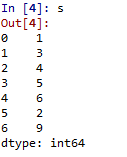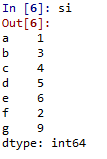### Create Pandas Series from Dictionary

Program to Create Pandas series from Dictionary.

## Python3

 `dictionary ``=` `{``'a'``: ``1``, ``'b'``: ``2``, ``'c'``: ``3``, ``'d'``: ``4``, ``'e'``: ``5``}`   `# Creating series of Dictionary type` `sd ``=` `pd.Series(dictionary)`

Output: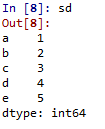Dictionary type data

### Convert an Array to Pandas Series

Program to Create ndarray series.

## Python3

 `# Defining 2darray` `Data ``=` `[[``2``, ``3``, ``4``], [``5``, ``6``, ``7``]]`   `# Creating series of 2darray` `snd ``=` `pd.Series(Data)`

Output: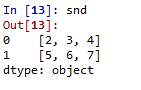Data as Ndarray

## Pandas DataFrames

The DataFrames in Pandas is a two-dimensional (2-D) data structure defined in pandas which consists of rows and columns.

## Python3

 `# Program to Create DataFrame`   `# Import Library` `import` `pandas as pd`   `# Create DataFrame with Data` `a ``=` `pd.DataFrame(Data)`

Here, Data can be:

1. One or more dictionaries
2. One or more Series
3. 2D-numpy Ndarray

### Create a Pandas DataFrame from multiple Dictionary

Program to Create a Dataframe with two dictionaries.

## Python3

 `# Define Dictionary 1` `dict1 ``=` `{``'a'``: ``1``, ``'b'``: ``2``, ``'c'``: ``3``, ``'d'``: ``4``}`   `# Define Dictionary 2` `dict2 ``=` `{``'a'``: ``5``, ``'b'``: ``6``, ``'c'``: ``7``, ``'d'``: ``8``, ``'e'``: ``9``}`   `# Define Data with dict1 and dict2` `Data ``=` `{``'first'``: dict1, ``'second'``: dict2}`   `# Create DataFrame` `df ``=` `pd.DataFrame(Data)`   `df`

Output: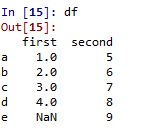DataFrame with two dictionaries

### Convert list of dictionaries to a Pandas DataFrame

Here, we are taking three dictionaries and with the help of from_dict() we convert them into Pandas DataFrame.

## Python3

 `import` `pandas as pd` `data_c ``=` `[` ` ``{``'A'``: ``5``, ``'B'``: ``0``, ``'C'``: ``3``, ``'D'``: ``3``},` ` ``{``'A'``: ``7``, ``'B'``: ``9``, ``'C'``: ``3``, ``'D'``: ``5``},` ` ``{``'A'``: ``2``, ``'B'``: ``4``, ``'C'``: ``7``, ``'D'``: ``6``}]`   `pd.DataFrame.from_dict(data_c, orient``=``'columns'``)`

Output:

```    A    B    C    D
0    5    0    3    3
1    7    9    3    5
2    2    4    7    6```

### Create DataFrame from Multiple Series

Program to create a dataframe of three Series.

## Python3

 `import` `pandas as pd`   `# Define series 1` `s1 ``=` `pd.Series([``1``, ``3``, ``4``, ``5``, ``6``, ``2``, ``9``])`   `# Define series 2    ` `s2 ``=` `pd.Series([``1.1``, ``3.5``, ``4.7``, ``5.8``, ``2.9``, ``9.3``])`   `# Define series 3` `s3 ``=` `pd.Series([``'a'``, ``'b'``, ``'c'``, ``'d'``, ``'e'``])    `   `# Define Data` `Data ``=``{``'first'``:s1, ``'second'``:s2, ``'third'``:s3}`   `# Create DataFrame` `dfseries ``=` `pd.DataFrame(Data)            `   `dfseries`

Output: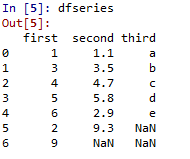DataFrame with three series

### Convert a Array to Pandas Dataframe

One constraint has to be maintained while creating a DataFrame of 2D arrays – The dimensions of the 2D array must be the same.

## Python3

 `# Program to create DataFrame from 2D array`   `# Import Library` `import` `pandas as pd`   `# Define 2d array 1` `d1 ``=``[[``2``, ``3``, ``4``], [``5``, ``6``, ``7``]]`   `# Define 2d array 2` `d2 ``=``[[``2``, ``4``, ``8``], [``1``, ``3``, ``9``]]`   `# Define Data` `Data ``=``{``'first'``: d1, ``'second'``: d2}`   `# Create DataFrame` `df2d ``=` `pd.DataFrame(Data)    `   `df2d`

Output: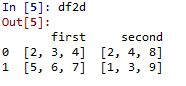DataFrame with 2d ndarray

Whether you're preparing for your first job interview or aiming to upskill in this ever-evolving tech landscape, GeeksforGeeks Courses are your key to success. We provide top-quality content at affordable prices, all geared towards accelerating your growth in a time-bound manner. Join the millions we've already empowered, and we're here to do the same for you. Don't miss out - check it out now!